Github - 基于CNN与三元组的图像检索实现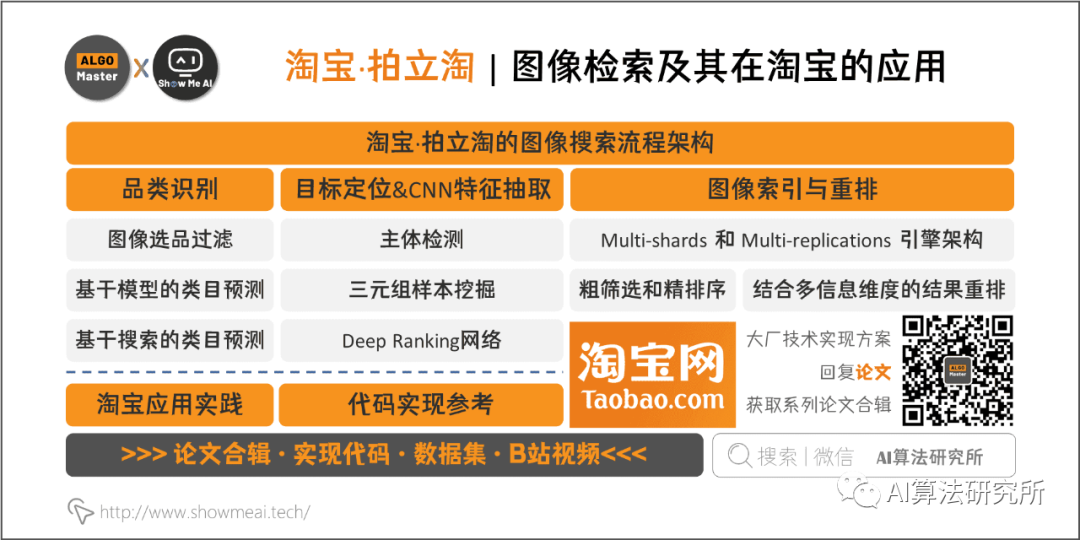## 1. 图像检索提升购物体验## 2. 淘宝·拍立淘的图像搜索流程架构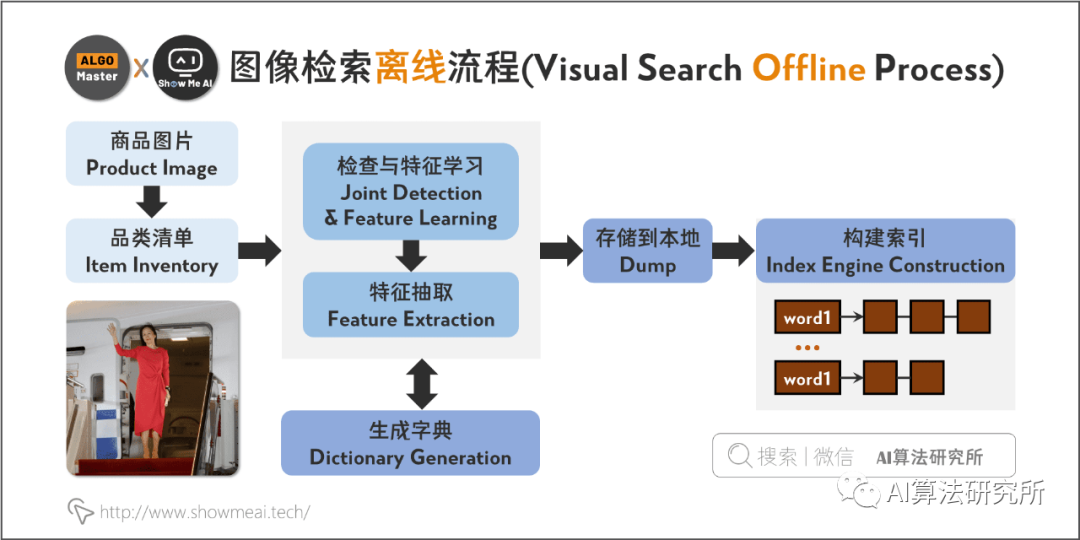### 2.1. 离线流程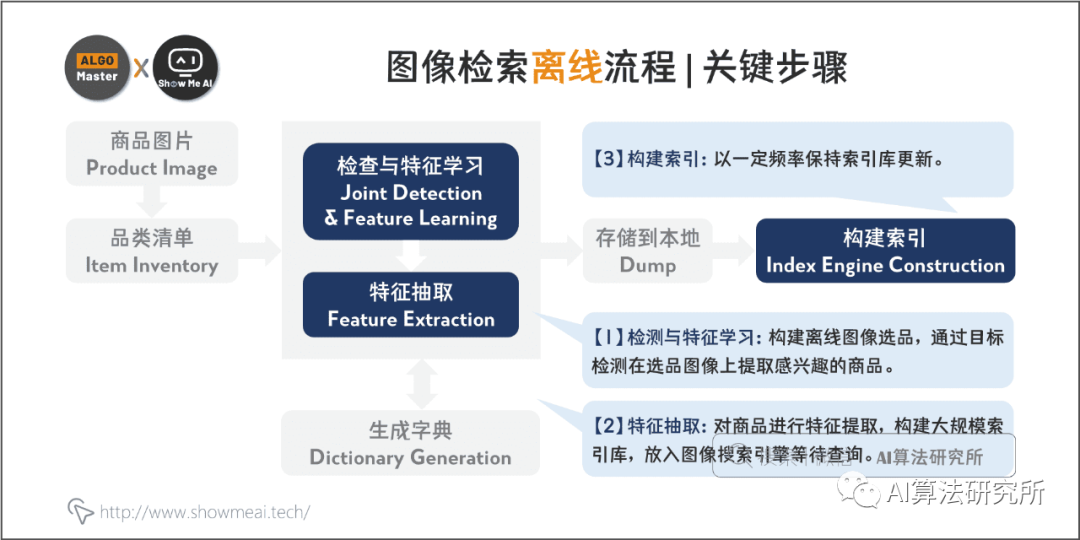- 检测与特征学习：构建离线图像选品，通过目标检测在选品图像上提取感兴趣的商品；

 - 特征抽取：对商品进行特征提取，构建大规模索引库，放入图像搜索引擎等待查询；

 - 构建索引：以一定频率保持索引库更新。

### 2.2. 在线流程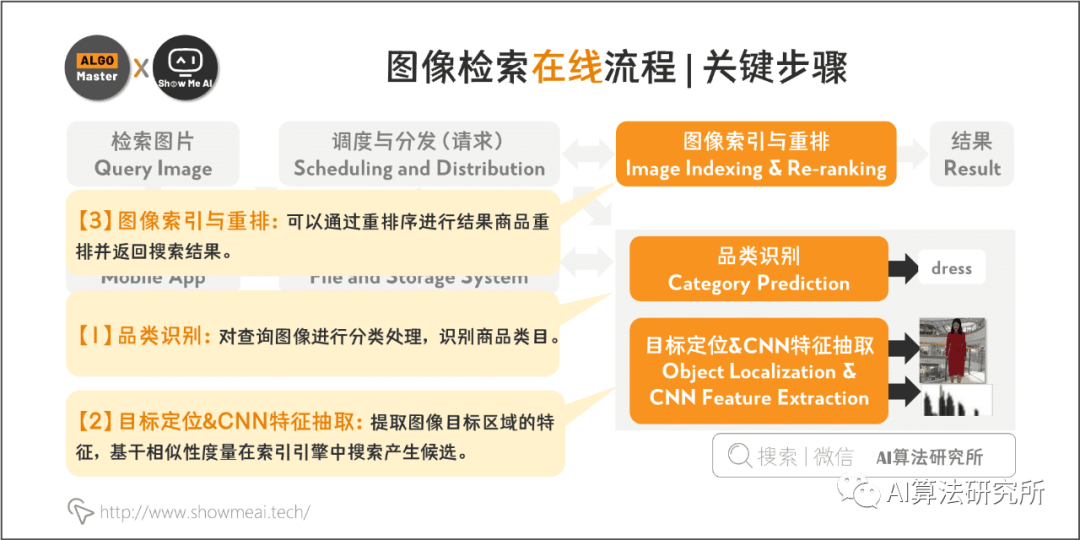- 品类识别：对查询图像进行分类处理，识别商品类目；

 - 目标定位 & CNN特征抽取：提取图像目标区域的特征，基于相似性度量在索引引擎中搜索产生候选；

 - 图像索引与重排：可以通过重排序进行结果商品重排并返回搜索结果。

## 3. 品类识别模块

### 3.1. 图像选品处理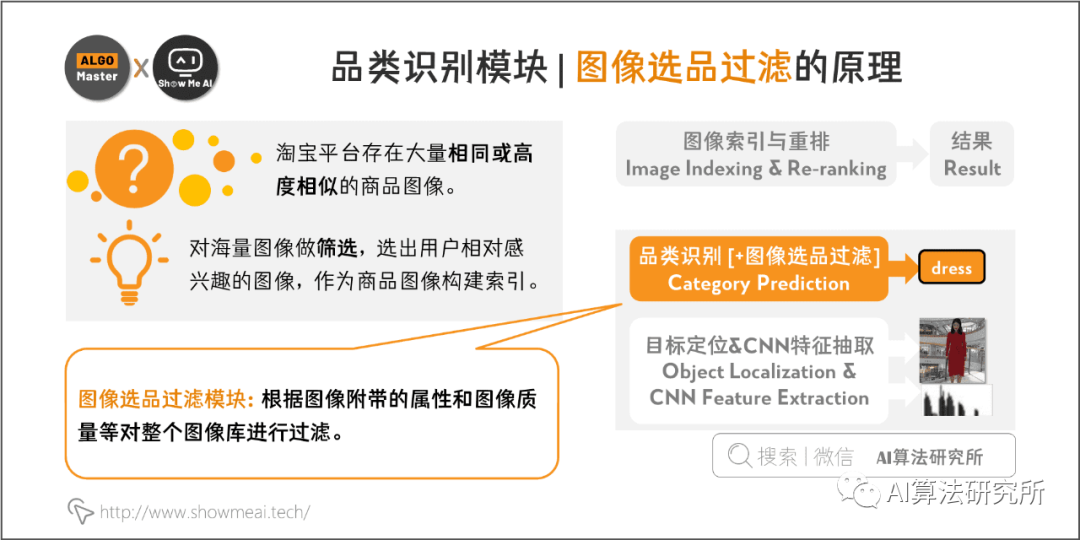### 3.2. 基于模型&搜索结合的类目预测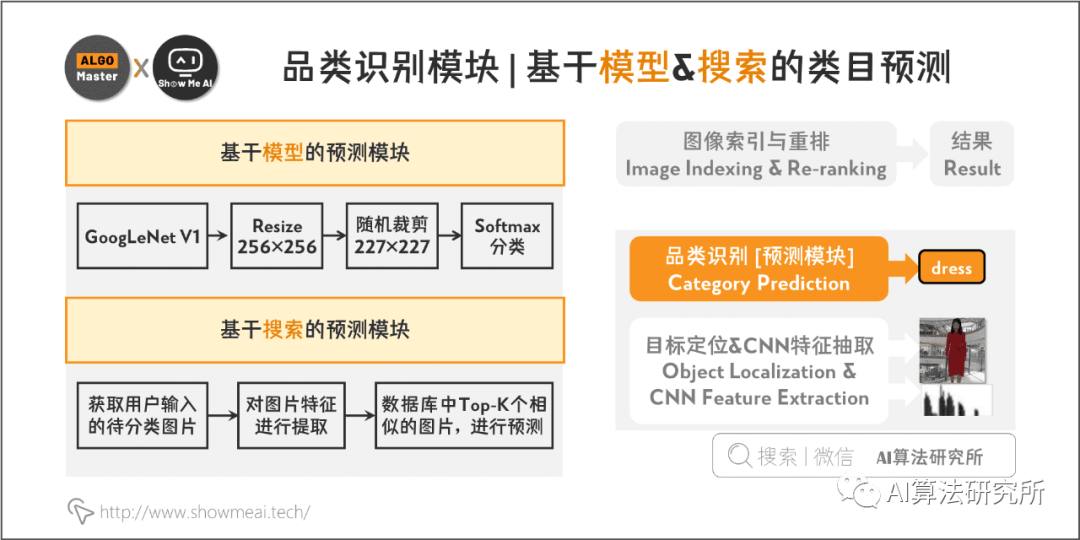#### 3.2.1. 基于模型的预测模块

 - 采用 GoogLeNet V1 网络结构来权衡高精度和低延迟，使用包含不同商品类目标签的图像集进行训练；

 - 将输入图像的大小 resize 为 256×256，随机裁剪到 227×227 ，使用 Softmax（交叉熵）损失函数作为分类任务的损失函数。

#### 3.2.2. 基于搜索的预测模块

 - 获取用户输入的待分类图片；

 - 应用基于搜索的分类方法：对图片特征提取，并在待检索的数据库中找出 Top-K 个和它相似的图片，根据这些图片的类目标签对输入图片进行预测。

#### 3.2.3. 淘宝·拍立淘应用实践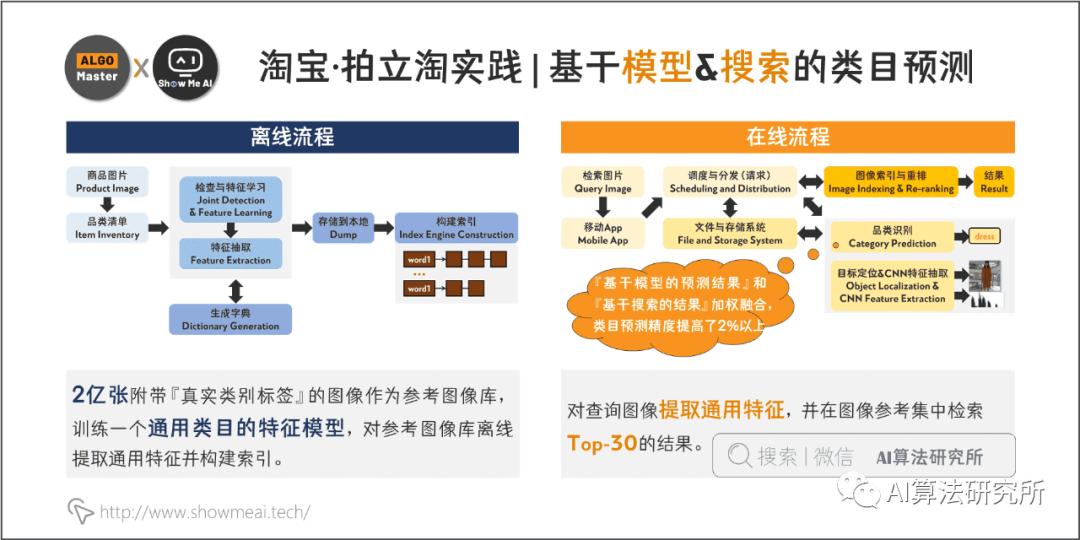• 离线部分

• 在线部分

• 品类识别的效果提升

## 4. 目标检测&特征联合学习&度量学习模块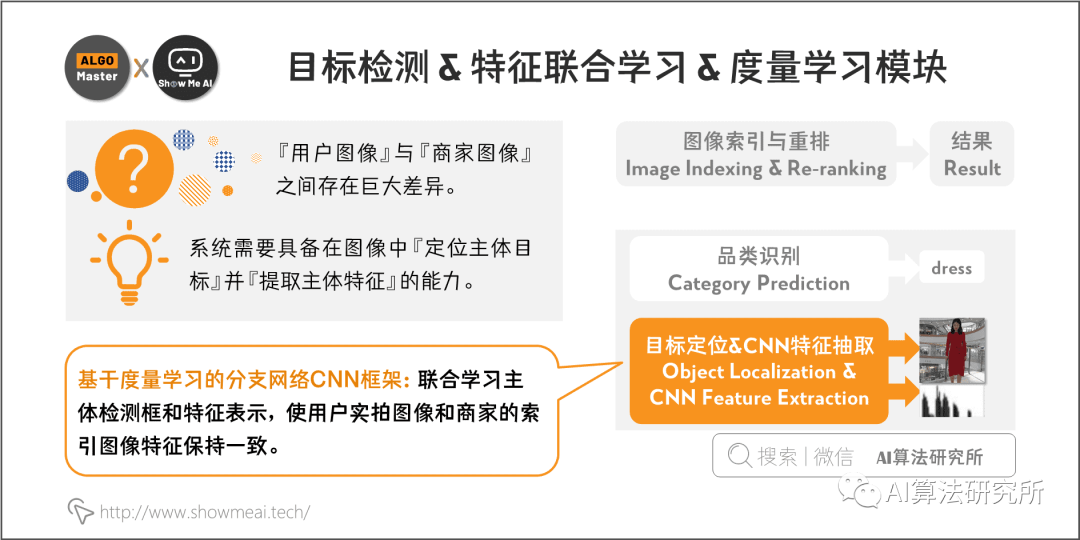• 第1行没有进行主体检测，检索结果明显受到了背景干扰；
• 第2行采用主体检测，检索结果有非常显著的效果提升。

### 4.1. 三元组挖掘

#### 4.1.1. 原理简介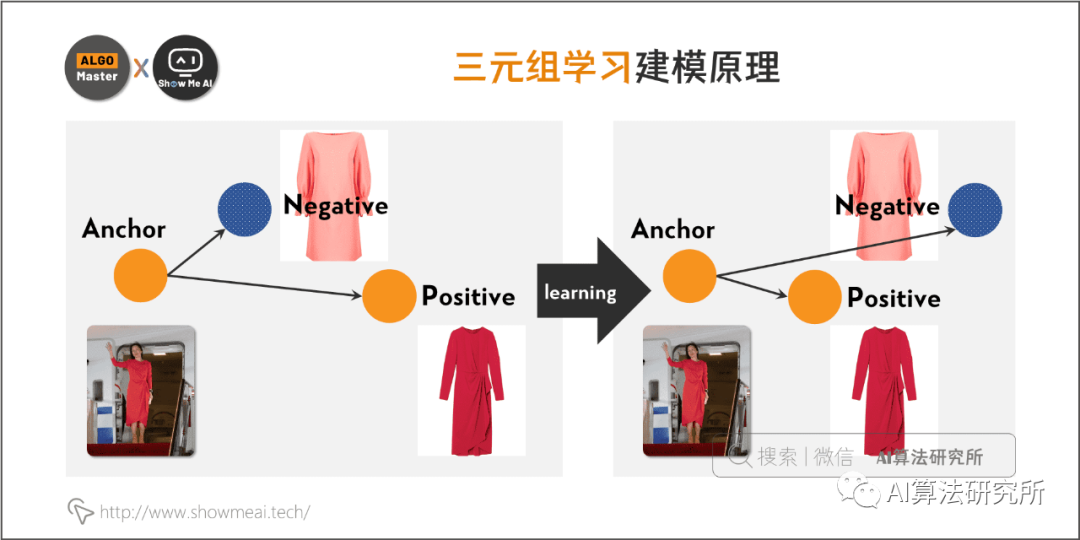Anchor 和 Positive 为同类的不同样本；

Anchor 与 Negative 为异类样本。

#### 4.1.2. 方法优缺点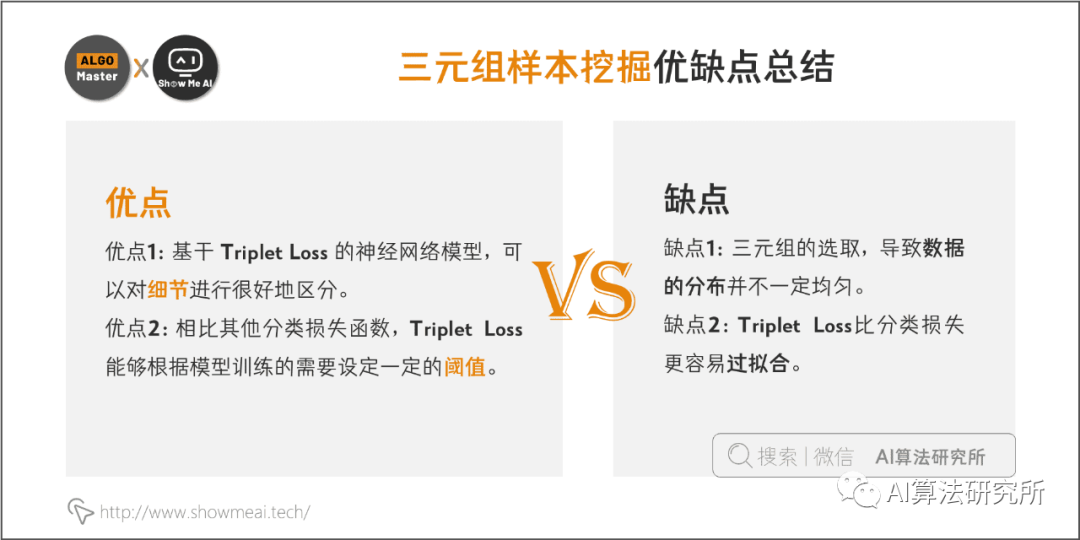- 优点1：基于 Triplet Loss 的神经网络模型，可以对细节进行很好地区分。

 - 优点2：相比其他分类损失函数，Triplet Loss 能够根据模型训练的需要设定一定的阈值。

 - 缺点1：三元组的选取，导致数据的分布并不一定均匀，所以在模型训练过程表现很不稳定且收敛慢，需要根据结果不断调节参数

 - 缺点2：Triplet Loss 比分类损失更容易过拟合。

#### 4.1.3. 淘宝拍立淘应用实践

$$loss(q, q^+, q^-) = [L_2(f(q), f(q^+)) - L_2(f(q), f(q^-)) + \delta ]_+$$

$L_2$ 表示两个向量之间的L2距离

$\delta$ 表示控制间隔margin 的参数

$f$ 表示需要学习的CNN特征提取器，可以通过端到端的训练学习到.

#### 4.1.4. 三元组样本挖掘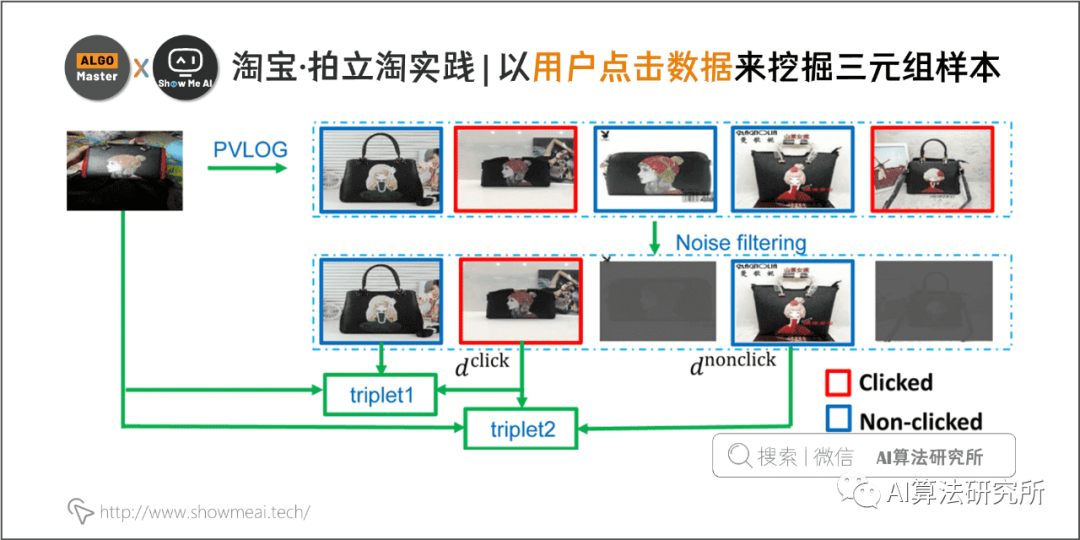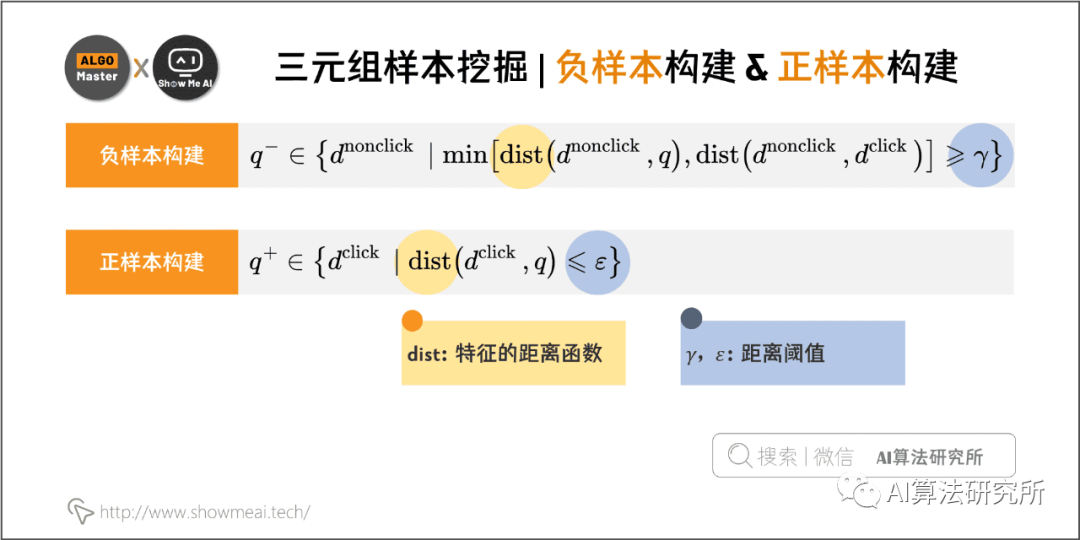$$q^- \in \lbrace d^{nonclick} | min [dist(d^{nonclick}, q), dist(d^{nonclick}, d^{click})] \geq \gamma \rbrace$$

$dist$ 为特征的距离函数

$\gamma$ 为距离阈值

$$q^+ \in \lbrace d^{click} | dist(d^{click}, q) \leq \epsilon \rbrace$$

 - 数据扩增

 - 损失函数优化

$$loss = \frac{1}{|Q|} \sum_{q \in Q} \frac{1}{|N_q|} \sum_{q^- \in N_q} [L_2 (f(q), f(q^+)) - L_2 (f(q), f(q^-)) + \delta]_+$$

$$Q = \lbrace q | \exists q^-, L_2(f(q), f(q^+)) - L_2(f(q), f(q^-)) + \delta > 0 \rbrace$$

$$N_q = \lbrace q^- | L_2(f(q), f(q^+)) - L_2(f(q), f(q^-)) + \delta > 0$$

#### 4.1.5. Triplet Loss 的简单代码实现示例

class TripletLoss(nn.Module):
"""
pytorch上的Triplet Loss实现
"""

def __init__(self, margin):
super(TripletLoss, self).__init__()
self.margin = margin

def forward(self, anchor, positive, negative, size_average=True):
distance_positive = (anchor - positive).pow(2).sum(1)  # .pow(.5)
distance_negative = (anchor - negative).pow(2).sum(1)  # .pow(.5)
losses = F.relu(distance_positive - distance_negative + self.margin)

return losses.mean() if size_average else losses.sum()

### 4.2. Deep Ranking 网络

#### 4.2.1. 网络结构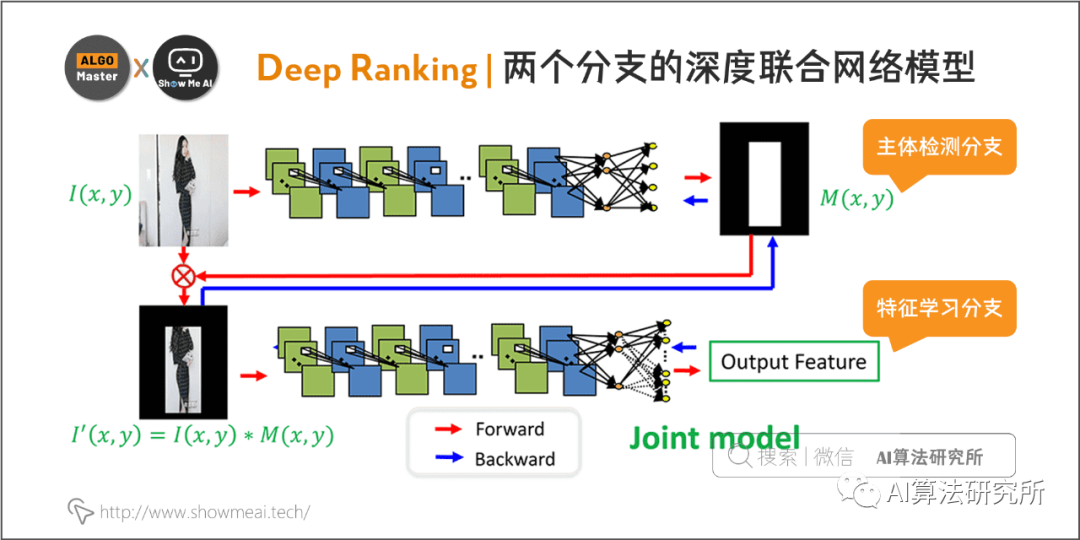#### 4.2.2. 参数学习与训练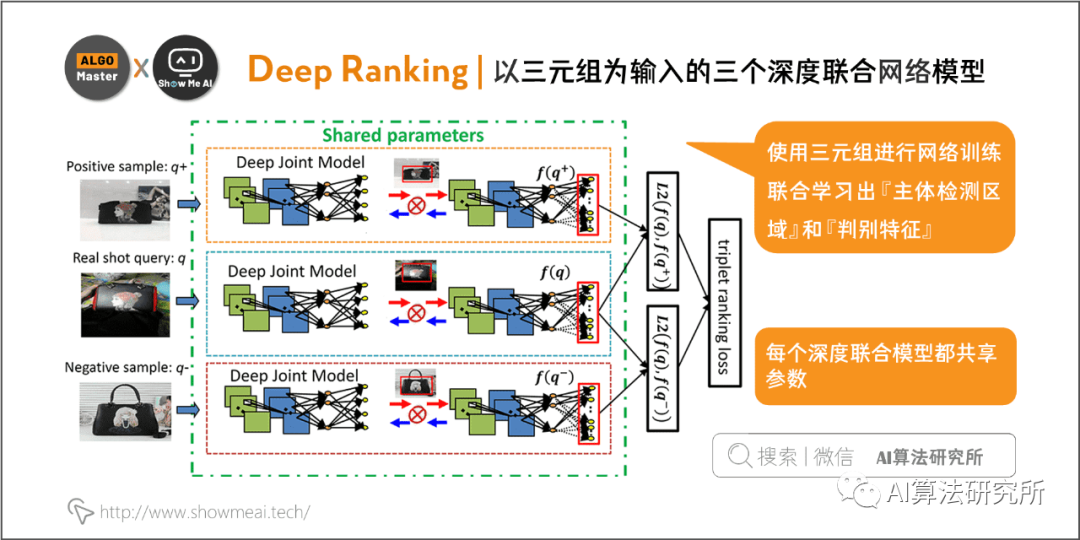Deep Ranking 框架下的每个深度联合模型都共享参数，检测的掩膜函数 $M(x,y)$ 如下公式所示，先利用检测分支回归出矩形坐标 $x_1, x_r, y_t, y_b$，再使用阶跃函数 $h$ 表示：

$$M(x, y) = [h(x-x_1) - h(x - x_r)] \times [h(y-y_t) - h(y - y_b)]$$

$$h(x-x_0) = \begin{cases} 0 & x < x_0 \\ 1 & x \geq x_0 \end{cases}$$

$$f(x) = \frac{1}{1 + e^{-kx}}$$

## 5. 图像索引与重排模块

Image Indexing & Re-ranking

### 5.1. 十亿级大规模图像检索引擎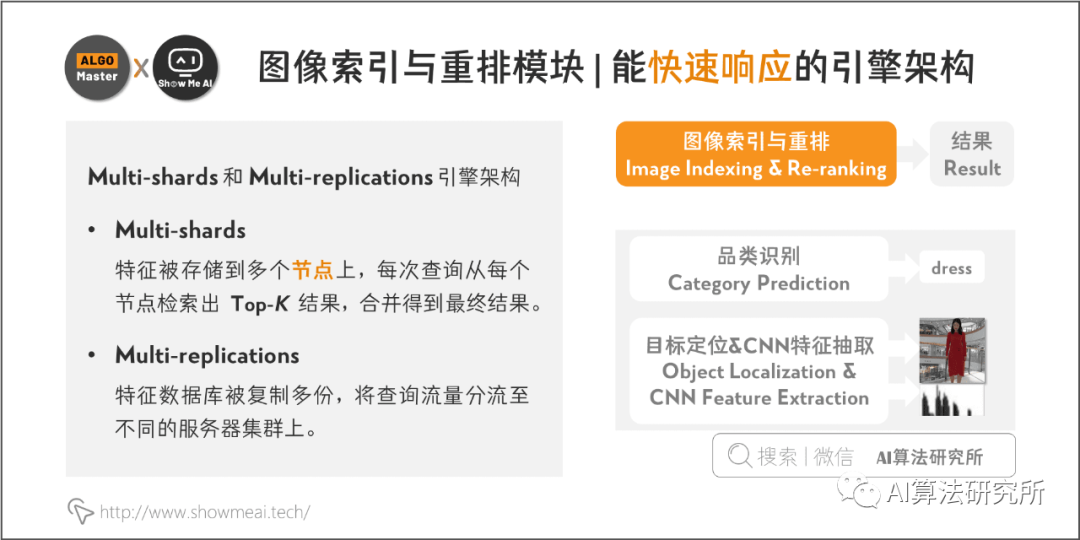#### 5.1.1. 结构框架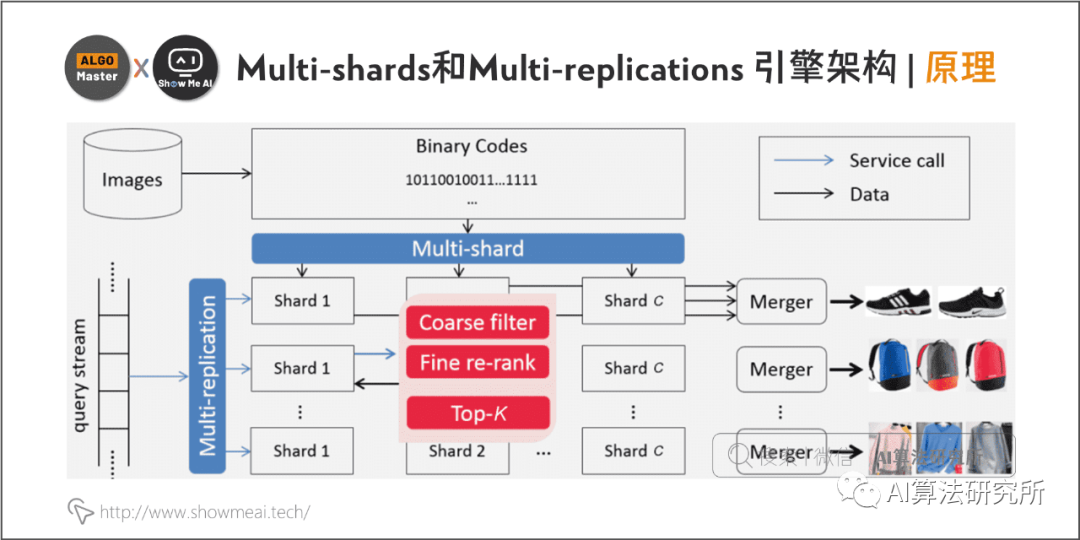• Multi-shards

• Multi-replications

#### 5.1.2. 粗筛选和精排序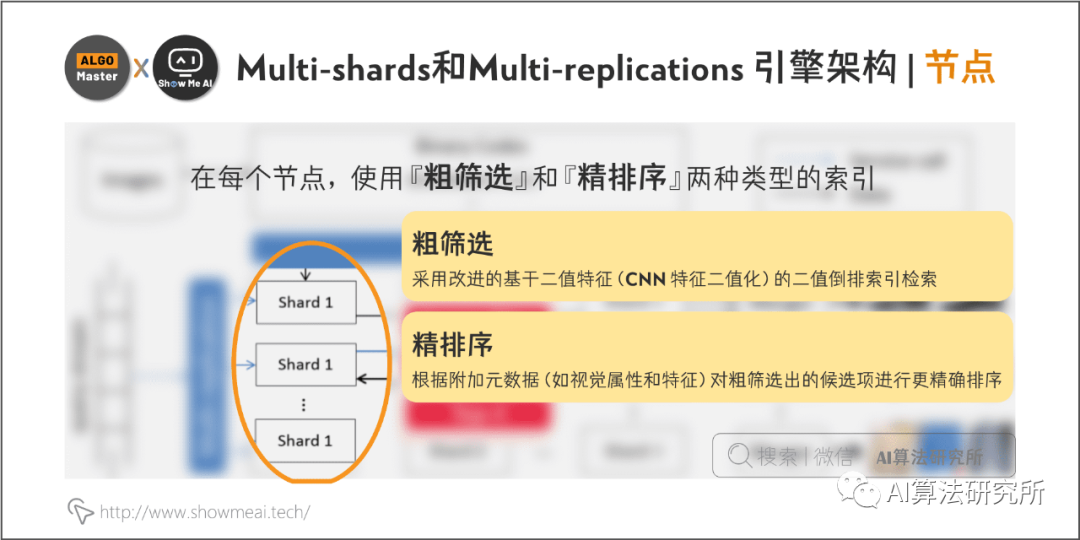- 粗筛选：采用改进的基于二值特征（CNN 特征二值化）的二值倒排索引检索。

1. 以图像ID为关键字、二值特征为值，通过汉明距离计算，快速滤除大量不匹配数据。
2. 根据返回的图像数据的二进制编码，对最近邻进行精排序。

 - 精排序：根据附加元数据（如视觉属性和特征）对粗筛选出的候选项进行更精确的排序。精排过程较慢：

1. 元数据以非二进制形式存储
2. 元数据的存储开销太大，无法将其全部载入内存中。这里缓存命中率是影响性能的关键因素。

### 5.2. 结合多信息维度的结果重排

#### 5.2.1. 存在的问题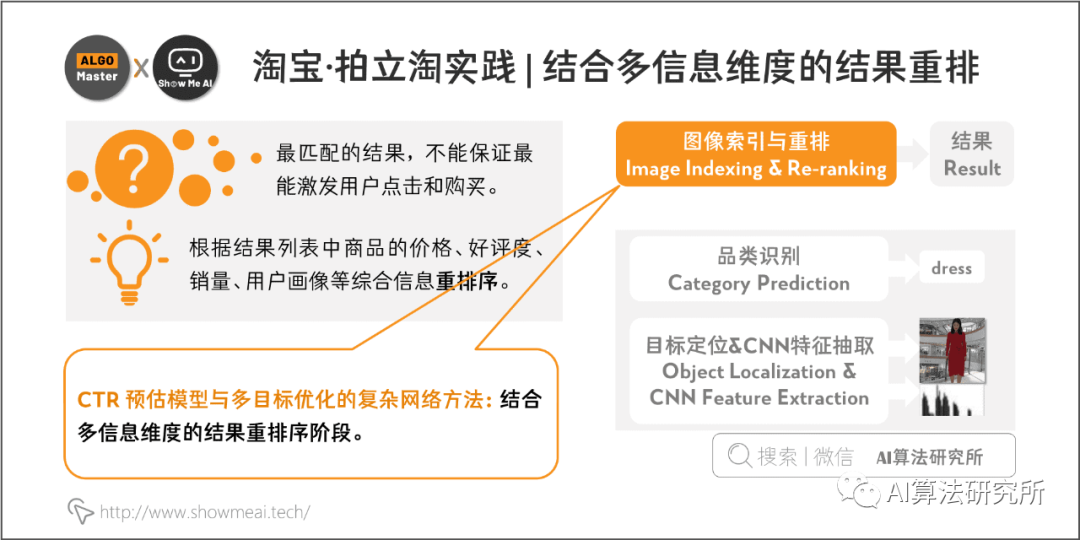## 参考文献

 Shaoqing Ren, Kaiming He, Ross B Girshick,et al. Faster R-CNN: Towards Real-Time Object Detection with Region ProposalNetworks. IEEE Transactions on Pattern Analysis and MachineIntelligence(T-PAMI), 2017:1137–1149.

 Wei Liu, Dragomir Anguelov, Dumitru Erhan,et al. SSD: Single Shot MultiBox Detector. In European Conference on ComputerVision (ECCV), 2016:21–37.

 YanhaoZhang, Pan Pan, Yun Zheng,et al. Visual Search at Alibaba. In Proceedings of the 24th InternationalConference on Knowledge Discovery and Data Mining (SIGKDD), 2018:993-1001

 Yushi Jing, David C Liu, Dmitry Kislyuk,et al. Visual Search at Pinterest. In Proceedings of the 21th InternationalConference on Knowledge Discovery and Data Mining (SIGKDD), 2015:1889–1898

 Christian Szegedy, Wei Liu, Yangqing Jia,et al. Going deeper with convolutions. In IEEE Conference on Computer Visionand Pattern Recognition(CVPR), 2015:1–9.

 Jiang Wang, Yang Song, Thomas Leung, etal. Learning Fine-Grained Image Similarity with Deep Ranking. In IEEEConference on Computer Vision and Pattern Recognition(CVPR), 2014:1386–1393.

 OlgaRussakovsky, Jia Deng, Hao Su, et al. ImageNet Large Scale VisualRecognition Challenge. (2014). arXiv:arXiv:1409.0575, 2014.

Last modification：June 2nd, 2022 at 01:21 am